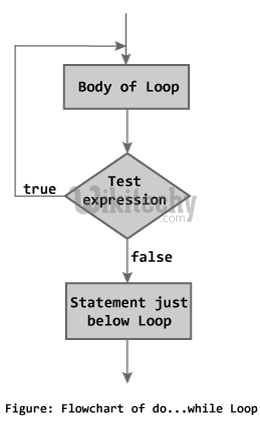# while Loop C++ - Learn C++ - C++ Tutorial - C++ programming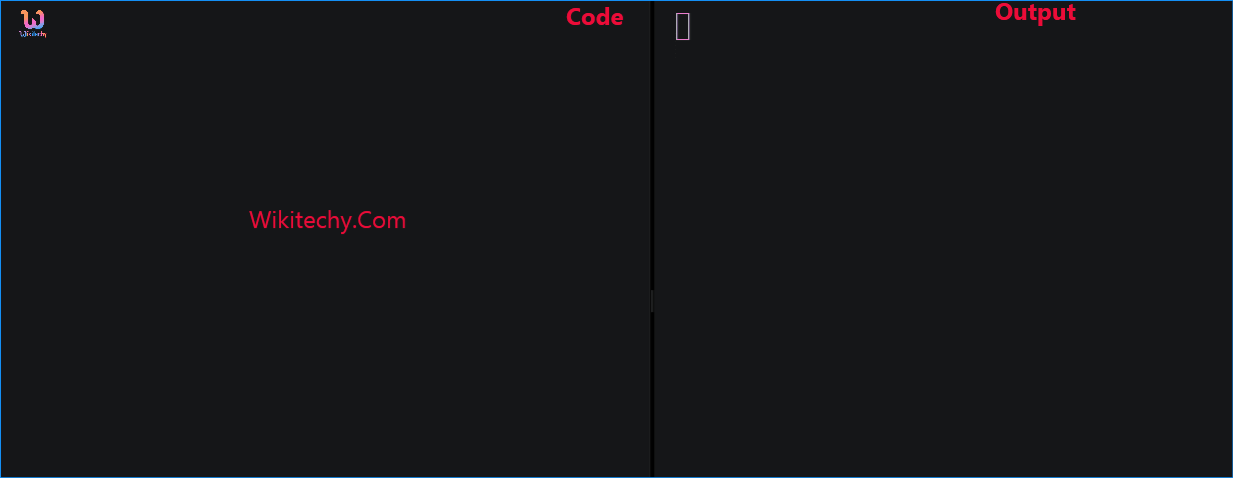Learn c++ - c++ tutorial - while loop c++ - c++ examples - c++ programs

## What is Loop in C++

• Loops are used in programming to repeat a specific block of code. In this article, you will learn to create while and do...while loops in C++ programming.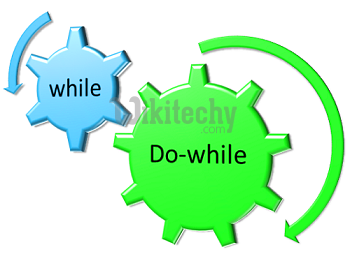• A loop statement allows us to execute a statement or group of statements multiple times
• In computer programming, loop repeats a certain block of code until some end condition is met.
• There are 3 type of loops in C++ Programming:
• for Loop
• while Loop
• do...while Loop
• In this we are going to explain while loop and do while loop

## C++ while Loop

• A while loop is a control flow statement that allows code to be executed repeatedly based on a given Boolean condition.
• The while loop evaluates the test expression.
• If the test expression is true, codes inside the body of while loop is evaluated.
• Then, the test expression is evaluated again. This process goes on until the test expression is false.
•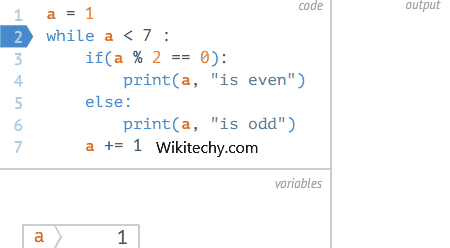learn c++ tutorials - while loop Example

• When the test expression is false, while loop is terminated.

## Syntax of a while loop

• where, testExpression is checked on each entry of the while loop.

## Flowchart of while Loop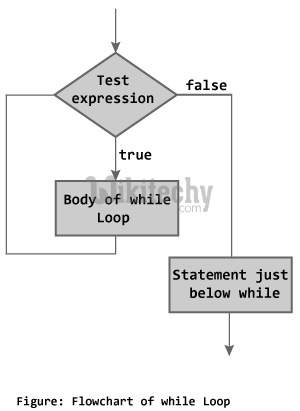## Output

• In this program, user is asked to enter a positive integer which is stored in variable number. Let's suppose, user entered 4.
• Then, the while loop starts executing the code. Here's how while loop works:
1. Initially, i = 1, test expression i <= number is true and factorial becomes 1.
2. Variable i is updated to 2, test expression is true, factorial becomes 2.
3. Variable i is updated to 3, test expression is true, factorial becomes 6.
4. Variable i is updated to 4, test expression is true, factorial becomes 24.
5. Variable i is updated to 5, test expression is false and while loop is terminated.

## C++ do...while Loop

• The do...while loop is a variant of the while loop with one important difference.
• The body of do...while loop is executed once before the test expression is checked.
• The codes inside the body of loop is executed at least once. Then, only the test expression is checked.
• If the test expression is true, the body of loop is executed. This process continues until the test expression becomes false.
• When the test expression is false, do...while loop is terminated

## Flowchart of do...while Loop